Foxtable(狐表)用户栏目专家坐堂 → 关于排考室座位号的问题

共有390人关注过本帖树形打印复制链接

# 主题：关于排考室座位号的问题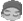1楼 | 信息 | 搜索 | 邮箱 | 主页 | UC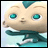加好友发短信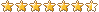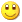Post By：2022/1/19 16:42:00 [显示全部帖子]

 ……CurrentTable.sort = "索引顺序"Dim k As Integer = 1Dim z As Integer = 1For b As Integer = 0 To CurrentTable.rows.count -1    CurrentTable.Rows(b)("考场号") = Format(k,"100")    CurrentTable.Rows(b)("座位号") = z    z = z + 1    If k<= 4        If z > 27 Then            z = 1            k = k + 1        End If    Else        If z > 26 Then            z = 1            k = k + 1        End If    End If    Next [此贴子已经被作者于2022/1/19 16:43:25编辑过]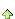2楼 | 信息 | 搜索 | 邮箱 | 主页 | UC加好友发短信Post By：2022/1/19 17:22:00 [显示全部帖子]

 For b As Integer = 0 To CurrentTable.rows.count -1    CurrentTable.Rows(b)("考场号") = Format(k,"100")    CurrentTable.Rows(b)("座位号") = z    z = z + 1        If z > 30 Then            z = 1            k = k + 1        End IfNext3楼 | 信息 | 搜索 | 邮箱 | 主页 | UC加好友发短信Post By：2022/1/19 17:38:00 [显示全部帖子]

 参考"考场号"的format用法呀，自己都用上了还不会？4楼 | 信息 | 搜索 | 邮箱 | 主页 | UC加好友发短信Post By：2022/1/20 8:31:00 [显示全部帖子]

 dim r as row = tables("表A").currentif r isnot nothing thenr("准考证号") = r("县级代号")+r("科类代号")+r("类别代号")+r("考生序号")end if5楼 | 信息 | 搜索 | 邮箱 | 主页 | UC加好友发短信Post By：2022/1/20 9:45:00 [显示全部帖子]

 CurrentTable.Rows(b)("考场号") = Format(k,"000")    CurrentTable.Rows(b)("座位号") = Format(z,"00") [此贴子已经被作者于2022/1/20 9:44:53编辑过]6楼 | 信息 | 搜索 | 邮箱 | 主页 | UC加好友发短信Post By：2022/1/20 10:48:00 [显示全部帖子]

 去掉红色代码，多余    For b As Integer = 0 To CurrentTable.rows.count -1        CurrentTable.Rows(b)("考场号") = Format(k,"100")  ''默认Format(k,"000")        CurrentTable.Rows(b)("座位号") = Format(Rand.Next(30) + b Mod e.Form.Controls("NumericComboBox1").Value,"00")        CurrentTable.Rows(b)("座位号") = z        CurrentTable.Rows(b)("座位号") = Format(z,"00")        z = z + 1        If z > 30 Then            z = 1            k = k + 1        End If    Next7楼 | 信息 | 搜索 | 邮箱 | 主页 | UC加好友发短信Post By：2022/1/20 11:23:00 [显示全部帖子]

 应该没有，测试再找问题了